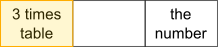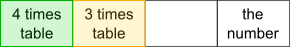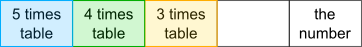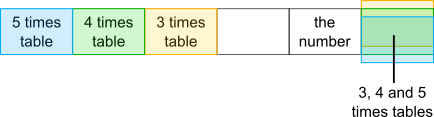#### You may also like### Consecutive Numbers

An investigation involving adding and subtracting sets of consecutive numbers. Lots to find out, lots to explore.### Calendar Capers

Choose any three by three square of dates on a calendar page...### Days and Dates

Investigate how you can work out what day of the week your birthday will be on next year, and the year after...

# One Short

##### Age 11 to 14 ShortChallenge LevelRemainder 2 when divided by 3Remainder 3 when divided by 4Remainder 4 when divided by 5The next number is 60
The number is 59
59$\div$7 = 8 remainder 3

(Note that the Chinese Remainder Theorem provides a general method for solving problems like this.)

This problem is taken from the UKMT Mathematical Challenges.
You can find more short problems, arranged by curriculum topic, in our short problems collection.